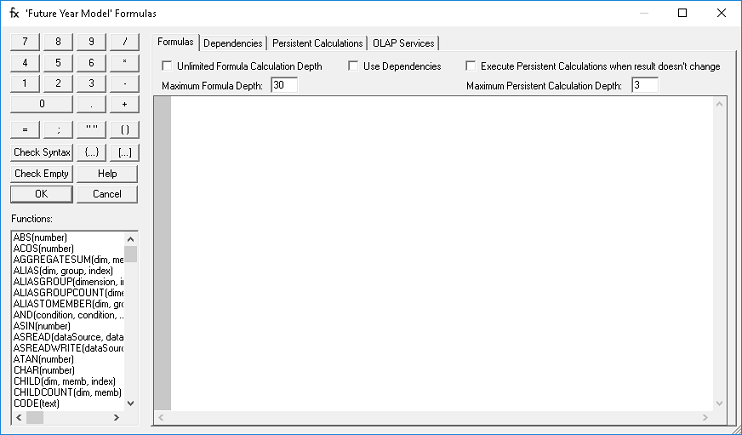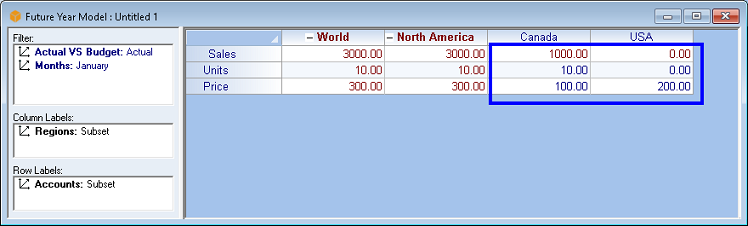Formula EngineA Formula Engine is a model whose calculations are triggered by a formula entered into the Cube. This model is more complex than the Pure Aggregation Cube. (Formula Editor below)Using the Formula Engine, there is a slower performance calculation due to the fact that the Formula language is extremely flexible. Given the formula language, you can have values at any location in the entire space of the Cube. We can’t make any assumptions about what the user may do with the formula language. If there is a Formula residing in the Formula Editor that can apply to any intersection within the Cube, the PowerOLAP® cannot skip or exclude calculation of any Detail members because there may be a value or a formulated value that governs a point somewhere in the Cube.

In the given example we have a Slice containing values for the Members Units and Price for both Canada and USA regions. As per the illustration, there are 10 Units for a Price of 100 per unit for the Region Canada and there are 0 units for a Price of 200 per unit for the Region USA. Our objective here is to calculate for the Sales value for the Regions Canada and USA.To calculate for the Sales value for the Regions Canada and USA we must first write a formula that will describe how to get or to compute for the Sales value. We will apply the following formula to get the Sales values:

 Sales = Unit x Price

Writing the formula in the Formula Editor:

1.   In the PowerOLAP ribbon, select Model tab and Cubes command.
The Cubes dialog box appears.

2.   Select a Cube (in the example the Future Year Model cube was selected)
then click on Formulas button to access the Formula Editor dialog.

3.   In the Formula Editor, go to the Formulas tab then write a formula.

In the example we will compute for the value of Sales by multiplying Unit by Price. This will be written in the Formula Editor screen in this manner:4.   Click on Check Syntax button to check for syntax errors.
A message box will prompt the screen indicating if there are syntax errors or none in the formula written.5.   If your formulas are free from any syntax errors, select OK button.
This will return you to the Cube dialog box.

6.   Back in the  Cubes dialog box, click OK.